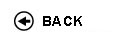Politecnico di Torino
Academic Year 2009/10
01AAWDR, 01AAWAX, 01AAWJA
Linear Algebra
1st degree and Bachelor-level of the Bologna process in Mechanical Engineering - Vercelli
1st degree and Bachelor-level of the Bologna process in Civil Engineering - Vercelli
1st degree and Bachelor-level of the Bologna process in Electronic And Computer Engineering - Vercelli
 Teacher Status SSD Les Ex Lab Tut Years teaching Ferrarotti MassimoAC MAT/03 30 20 0 20 11
 SSD CFU Activities Area context MAT/02 5 A - Di base Matematica, informatica e statistica
 Objectives of the course The goal of this course is to learn the fundamental tools of linear algebra which are basic for engineering applications. Expected skills At the end of the course the student will be able to use matrix calculus, to solve linear systems, to compute dimension, bases, intersections and unions of vector and subvector spaces, to compute eigenvalues, eigenvectors with applications to diagonalization of square matrices and to the study of conics in the plane. Prerequisites The students are expected to know results and techniques from trigonometry, complex numbers and basic mathematics of high school. Syllabus - Matrices (sum, products, rank, determinant, invertible matrices). - Linear systems (Gauss algorithm , Rouch�-Capelli's Theorem, Cramer's Theorem). - Vector spaces (geometrical meaning, basis, dimension, subspaces: intersection sum and direct sum). - Linear applications (Kernel, images, composition). - Eigenvalues and eigenvectors , similarity to diagonal matrices. - Euclidean spaces (norm for vectors, orthonormal basis). Spectral Theorem and quadratic forms. - Geometry in coordinates of the plane (line and conics). Laboratories and/or exercises Exercise sections are done during the course. Bibliography M. Ferrarotti, Appunti di Algebra lineare , Ed. Otto. Saranno disponibili in rete dispense del docente. M.L. Spreafico, Esercizi relativi al corso di Algebra lineare , disponibili presso Politeko. Testi di consultazione e di esercizi: N. Chiarli, S. Greco, P. Valabrega, 100 esercizi di... Algebra Lineare, ed. Levrotto e Bella. N. Chiarli, S. Greco, P. Valabrega, 100 esercizi di... Geometria analitica piana, ed. Levrotto e Bella. S.Greco, P.Valabrega, Lezioni di Geometria, ed. Levrotto e Bella. A.Sanini, Elementi di Geometria, Levrotto e Bella. Revisions / Exam The examination consists in a written proof based on resolution of exercises and in an oral discussion about the subjects contained in the program. No calculator, no texts or notes can be used during the examination. Programma definitivo per l'A.A.2009/10© Politecnico di Torino
Corso Duca degli Abruzzi, 24 - 10129 Torino, ITALY